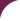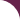# Ninth Grade Curriculum

Math
Algebra II

Algebra II is also known as Advanced Algebra. In this course we will discussing topics already learned in Algebra I in a more advance level. These topics include systems of equations and inequalities, quadratic functions, radical functions and rational functions. We will also discuss new topics such as conic sections, sequences and series, trigonometric functions, trigonometric identities and equations and statistics and probability.